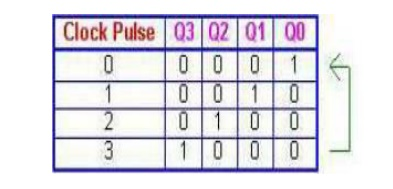# Counters

In electronics, counters can be implemented quite easily using register-type circuits such as the flip-flop, and a wide variety of designs exist, ü Asynchronous (ripple) counters ü Synchronous counters ü Johnson counters ü Decade counters ü Up-Down counters ü Ring counters

COUNTERS

In digital logic and computing, a counter is a device which stores (and sometimes displays) the number of times a particular event or process has occurred, often in relationship to a clock signal. In practice, there are two types of counters:

·        up counters which increase (increment) in value

·        down counters which decrease (decrement) in value

Counters Types

In electronics, counters can be implemented quite easily using register-type circuits such as the flip-flop, and a wide variety of designs exist,

ü Asynchronous (ripple) counters

ü Synchronous counters

ü Johnson counters

ü Up-Down counters

ü Ring counters

Each is useful for different applications. Usually, counter circuits are digital in nature, and count in binary, or sometimes binary coded decimal. Many types of counter circuit are available as digital building blocks, for example a number of chips in the 4000 series implement different counters.

ü Asynchronous (ripple) counters

The simplest counter circuit is a single D-type flip flop, with its D (data) input fed from its own inverted output. This circuit can store one bit, and hence can count from zero to one before it overflows (starts over from 0). This counter will increment once for every clock cycle and takes two clock cycles to overflow, so every cycle it will alternate between a transition from 0 to 1 and a transition from 1 to 0. Notice that this creates a new clock with a 50% duty cycle at exactly half the frequency of the input clock. If this output is then used as the clock signal for a similarly arranged D flip flop (remembering to invert the output to the input), you will get another 1 bit counter that counts half as fast. Putting them together yields a two bit counter:

ü Synchronous counters

Where a stable count value is important across several bits, which is the case in most counter systems, synchronous counters are used. These also use flip-flops, either the D-type or the more complex J-K type, but here, each stage is clocked simultaneously by a common clock signal. Logic gates between each stage of the circuit control data flow from stage to stage so that the desired count behavior is realized. Synchronous counters can be designed to count up or down, or both according to a direction input, and may be presetable via a set of parallel "jam" inputs. Most types of hardware-based counter are of this type.

A simple way of implementing the logic for each bit of an ascending counter (which is what is shown in the image to the right) is for each bit to toggle when all of the less significant bits are at a logic high state. For example, bit 1 toggles when bit 0 is logic high; bit 2 toggles when both bit 1 and bit 0 are logic high; bit 3 toggles when bit 2, bit 1 and bit 0 are all high; and so on.

ü Johnson counters

A Johnson counter is a special case of shift register, where the output from the last stage is inverted and fed back as input to the first stage. A pattern of bits equal in length to the shift register thus circulates indefinitely. These counters are sometimes called "walking ring" counters, and find specialist applications, including those similar to the decade counter, digital to analogue conversion, etc.The apparent disadvantage of this counter is that the maximum available states are not fully utilized. Only eight of the sixteen states are being used.

Decade counters are a kind of counter that counts in tens rather than having a binary representation. Each output will go high in turn, starting over after ten outputs have occurred. This type of circuit finds applications in multiplexers and demultiplexers, or wherever a scanning type of behaviour is useful. Similar counters with different numbers of outputs are also common.

ü Up-Down Counters

It is a combination of up counter and down counter, counting in straight binary sequence. There is an up-down selector. If this value is kept high, counter increments binary value and if the value is low, then counter starts decrementing the count. The Down counters are made by using the complemented output to act as the clock for the next flip-flop in the case of Asynchronous counters. An Up counter is constructed by linking the Q out of the J-K Flip flop and putting it into a Negative Edge Triggered Clock input. A Down Counter is constructed by taking the Q output and putting it into a Positive Edge Triggered input

ü Ring Counters

A ring counter is basically a circulating shift register in which the output of the most significant stage is fed back to the input of the least significant stage. The following is a 4-bit ring counter constructed from D flip-flops. The output of each stage is shifted into the next stage on the positive edge of a clock pulse. If the CLEAR signal is high, all the flip -flops except the first one FF0 are reset to 0. FF0 is preset to 1 instead.Since the count sequence has 4 distinct states, the counter can be considered as a mod-4 counter. Only 4 of the maximum 16 states are used, making ring counters very inefficient in terms of state usage. But the major advantage of a ring counter over a binary counter is that it is self-decoding. No extra decoding circuit is needed to determine what state the counter is in.Applications of counters:

Watches

Clocks

Alarms

Web browser refresh

Study Material, Lecturing Notes, Assignment, Reference, Wiki description explanation, brief detail
Digital Principles and System Design : Synchronous Sequential Logic : Counters |